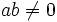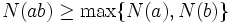Multiplicatively monotone norm

This article defines a property that can be evaluated for a norm on a commutative unital ring: a function from the nonzero elements of the ring to the integers.
View a complete list of properties of norms

Definition

A multiplicatively monotone norm on a commutative unital ring is a function from its nonzero elements to the nonnegative integers with the property that the norm of a product is at least equal to the norms of the factors.

In symbols, it is a function$N: R \setminus \{ 0 \} \to \mathbb{N}_0$ such that for$ab \ne 0$, we have:$N(ab) \ge \max \{ N(a), N(b) \}$.

This definition is typically used for integral domains.